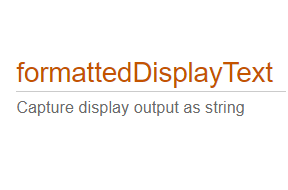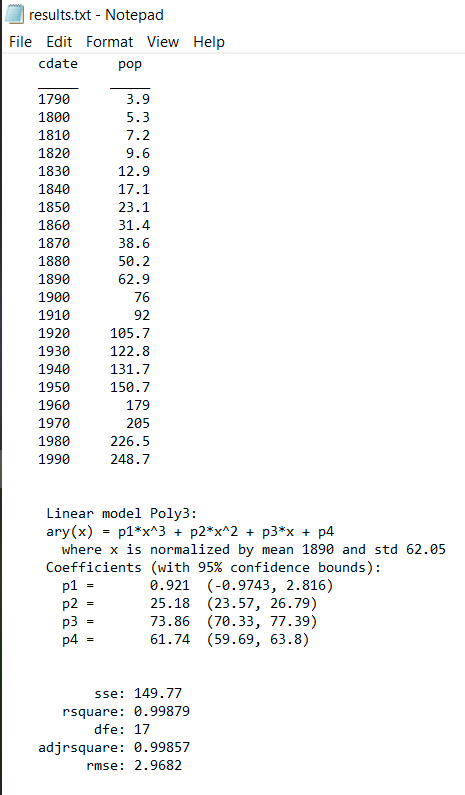# Community Highlights

### Community Pick

400 views (last 30 days)

## New in R2021a: Capture disp() output as a string

Adam Danz 2021년 3월 15일 (2021년 4월 29일에 수정됨)
Latest activity Edit by Hans Scharler 2021년 4월 29일

We've all been there. You've got some kind of output that displays perfectly in the command window and you just want to capture that display as a string so you can use it again somewhere else. Maybe it's a multidimensional array, a table, a structure, or a fit object that perfectly displays the information you need in a neat and tidy format but when you try to recreate the display in a string variable it's like reconstructing the Taj Mahal out of legos.Use str=formattedDisplayText(var) the same way you use disp(var) except instead of displaying the output, it's stored as a string as it would appear in the command window.

Additional name-value pairs allow you to

• Specify a numeric format
• Specify loose|compact line spacing
• Display true|false instead of 1|0 for logical values
• Include or suppress markup formatting that may appear in the display such as the bold headers in tables.

Demo: Record the input table and results of a polynomial curve fit

```load census
[fitobj, gof] = fit(cdate, pop, 'poly3', 'normalize', 'on')
```

Results printed to the command window:

```fitobj =
Linear model Poly3:
fitobj(x) = p1*x^3 + p2*x^2 + p3*x + p4
where x is normalized by mean 1890 and std 62.05
Coefficients (with 95% confidence bounds):
p1 =       0.921  (-0.9743, 2.816)
p2 =       25.18  (23.57, 26.79)
p3 =       73.86  (70.33, 77.39)
p4 =       61.74  (59.69, 63.8)
```
```gof =
struct with fields:
```
```             sse: 149.77
rsquare: 0.99879
dfe: 17
rmse: 2.9682```

Capture the input table, the printed fit object, and goodness-of-fit structure as strings:

```rawDataStr = formattedDisplayText(table(cdate,pop),'SuppressMarkup',true)
fitStr = formattedDisplayText(fitobj)
gofStr = formattedDisplayText(gof)
```

Display the strings:

```rawDataStr =
"    cdate     pop
_____    _____
1790       3.9
1800       5.3
1810       7.2
1820       9.6
1830      12.9
1840      17.1
1850      23.1
1860      31.4
1870      38.6
1880      50.2
1890      62.9
1900        76
1910        92
1920     105.7
1930     122.8
1940     131.7
1950     150.7
1960       179
1970       205
1980     226.5
1990     248.7
"
```
```fitStr =
"     Linear model Poly3:
ary(x) = p1*x^3 + p2*x^2 + p3*x + p4
where x is normalized by mean 1890 and std 62.05
Coefficients (with 95% confidence bounds):
p1 =       0.921  (-0.9743, 2.816)
p2 =       25.18  (23.57, 26.79)
p3 =       73.86  (70.33, 77.39)
p4 =       61.74  (59.69, 63.8)
"
```
```gofStr =
"           sse: 149.77
rsquare: 0.99879
dfe: 17
rmse: 2.9682
"
```

Combine the strings into a single string and write it to a text file in your temp directory:

```txt =  strjoin([rawDataStr; fitStr; gofStr],[newline newline]);
file = fullfile(tempdir,'results.txt');
fid = fopen(file,'w+');
cleanup = onCleanup(@()fclose(fid));
fprintf(fid, '%s', txt);
clear cleanup
```

Open results.txt.

```winopen(file) % for Windows platforms
```#### 3개의 댓글

Yvan Lengwiler 2021년 4월 20일

Well that seems a bit superfluous.

```str = evalc('disp(table(cdate,pop))');
```

And if you want to remove html tags from the output, do this:

```str = regexprep(str,'<.*?>','');
```

1개의 답글
Adam Danz 2021년 4월 22일 (2021년 4월 22일에 수정됨)

Almost... you need to wrap the output in a string to match the output of formattedDisplayText. Also, no need to call regexprep to remove the hotlinks and markup. You could do this instead,

```str = string(evalc('feature(''hotlinks'',''off'');disp(table(cdate,pop))'))
```

or, of course, just call this line which is a bit more readable IMO

```formattedDisplayText(table(cdate,pop),'SuppressMarkup', true)
```

formattedDisplayText has some other features that set it apart from using a simple evalc.

Let's look at a table of exchange rates with bank number formatting

```c = {'USD', 1; 'EURO', 0.830213; 'Pound', 0.716957; 'Rupee', 75.299172};
string(evalc('disp(c)'))
formattedDisplayText(c,'NumericFormat','bank')
```
```|evalc                          formattedDisplayText
"    {'USD'  }    {[      1]}   "    {'USD'  }    {[ 1.00]}
{'EURO' }    {[0.83021]}        {'EURO' }    {[ 0.83]}
{'Pound'}    {[0.71696]}        {'Pound'}    {[ 0.72]}
{'Rupee'}    {[ 75.299]}        {'Rupee'}    {[75.30]}
"                               "|
```

or displaying values as fractions or ratios

```r = randi(20,1,5)./randi(40,1,5);
string(evalc('disp(r)'))
formattedDisplayText(r,'NumericFormat','rational')
```
```evalc
"  0.35294   0.42857   0.025   0.67857   0.71429
"
formattedDisplayText
"  6/17      3/7       1/40    19/28      5/7
"
```

How about differentiating between numeric and logical values?

```b = num2cell(randi(2,5,5)-1);
ri = randperm(25,12);
b(ri(1:6)) = {false};
b(ri(7:12)) = {true};
string(evalc('disp(b)'))
formattedDisplayText(b,'UseTrueFalseForLogical',true)
```
```evalc:
"    {}    {}    {}    {}    {}
{}    {}    {}    {}    {}
{}    {}    {}    {}    {}
{}    {}    {}    {}    {}
{}    {}    {}    {}    {}
"
formattedDisplayText:
"    {[    0]}    {[   0]}    {[   1]}    {[    1]}    {[false]}
{[false]}    {[   1]}    {[true]}    {[false]}    {[    0]}
{[false]}    {[true]}    {[   0]}    {[    1]}    {[    0]}
{[false]}    {[true]}    {[   0]}    {[    1]}    {[false]}
{[ true]}    {[true]}    {[true]}    {[    1]}    {[    1]}
"
```

Of course, all of these options can be implemented using evalc with a bit of finesse. In fact, formattedDisplayText uses evalc and should come with the same security warning in the evalc documentation .

goc3 2021년 3월 15일

This seems like a reliable alternative to diary, which doesn't always turn on and off when intended. Thanks for pointing this out, Adam.

### Community Treasure Hunt

Find the treasures in MATLAB Central and discover how the community can help you!

Start Hunting!